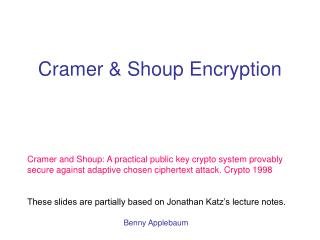DownloadDownload PresentationCramer & Shoup Encryption

# Cramer & Shoup Encryption

Download Presentation## Cramer & Shoup Encryption

- - - - - - - - - - - - - - - - - - - - - - - - - - - E N D - - - - - - - - - - - - - - - - - - - - - - - - - - -
##### Presentation Transcript

1. Cramer & Shoup Encryption Cramer and Shoup: A practical public key crypto system provably secure against adaptive chosen ciphertext attack. Crypto 1998 These slides are partially based on Jonathan Katz’s lecture notes. Benny Applebaum

2. Generate (PK,SK) PK DSK(c1) DSK(cp) b{0,1} C=EPK(mb) CCA1 Security A c1 cp (m0,m1) b’ A wins if b=b’. The scheme is CCA1 secure if any efficient A wins with probability <1/2+neg

3. DDH Assumption • Let G be a cyclic group of (prime) order q • DH tuple: (g,ga,gb,gab) • Rand tuple (g,ga,gb,gc) • where g is a random generator and a,b,cZq • DDH Assumption: Hard to distinguish Rand from DDH • |Pr[A(DH)=1]-Pr[A(Rand)=1]|<negl, for any poly-time A

4. Cramer & Shoup Lite • PK= (g1,g2,h=g1xg2y, c= g1ag2b) • g1,g2 are random generators and x,y,a,bZq • SK = (x,y,a,b) • EPK(m): choose r Zq; set C=(g1r,g2r, hr m, cr) • DSK(u,v,w,e): • If euavb then output  • Else, output w/(uxvy)

5. Cramer & Shoup Lite • PK= (g1,g2,h=g1xg2y, c= g1ag2b) • g1,g2 are random generators and x,y,a,bZq • SK = (x,y,a,b) • EPK(m): choose r Zq; set C=(g1r,g2r, hr m, cr) • DSK(u,v,w,e): • If euavb then output  • Else, output w/(uxvy) • Correctness: Easy…

6. x,y,a,bZq; SK=(x,y,a,b) PK= (g1,g2,h=g1xg2y, c= g1ag2b) DSK(c1) DSK(cp) b{0,1} C=(g3 ,g4, g3xg4y mb, g3ag4b) CSL is CCA1 secure • Assume that A breaks CSL via CCA1 • Construct A’ that breaks DDH A’ (g1,g2,g3,g4) A c1 cp (m0,m1) b’ If b=b’ then output “DDH” otherwise output “Rand”

7. CSL is CCA1 secure Thm. Under the DDH, CSL is CCA1 secure. Proof: • |Pr[A’(DH)=1]-Pr[A’(Rand)=1]|<negl follows from DDH Assum. and since A’ is poly-time • Claim: Pr[A’=1|DH]=Pr[A CCA1 breaks CSL] • Claim: |Pr[A’=1|Rand]| ½ + negl Hence: Pr[A CCA1 breaks CSL] =Pr[A’=1|DH]  |Pr[A’=1|Rand]|+negl  1/2+negl

8. CSL is CCA1 secure Claim 3: |Pr[A’=1|Rand]| ½ + negl Proof: • Show that (except w/neg prob) A attacks a perfect cipher. • I.e, g3xg4y is random (according to A’s view). • Let (g1,g2 = g1,g3 = g1r ,g4 = g1 r’) • Except w/neg prob 0,rr’ • From PK, A knows h=g1xg2y;that is, logg1 h=x+y (*) • We saw: if A knows only (*) then g3xg4y is random (from A’s view). Lemma: in phase 2 (except w/neg prob) A doesn’t learn info regarding (x,y). Proof: • A query (u,v,w,e) is bad if logg1 u  logg2 v and DSK(u,v,w,e)   Claim 4: (except w/neg prob) A’s queries are all good Claim 5: If A’s queries are all good then A does not learn additional info regarding (x,y) in phase 2

9. CSL is CCA1 secure • Is CSL CCA2 secure? • Why the argument fail to prove CCA2 security?

10. Generate (PK,SK) PK DSK(c1) DSK(cp) b{0,1} C*=EPK(mb) DSK(c1) DSK(cp) CCA2 Security A c1 cp (m0,m1) c’1c* c’p c* b’ A wins if b=b’. The scheme is CCA2 secure if any efficient A wins with probability <1/2+neg

11. The Cramer & Shoup Cryptosystem • PK= (g1,g2,h=g1xg2y, c= g1ag2b , d= g1a’g2b’,H) g1,g2 are random generators, x,y,a,b,a’,b’Zq and H is a hash function • SK = (x,y,a,b,a’,b’) • EPK(m): choose r Zq; set C=(g1r,g2r, hr m, (cd)r), where =H(g1r,g2r, hr m) • DSK(u,v,w,e): • If eua + a’vb+ b’ (where =H(g1r,g2r, hr m)) then output  • Else, output w/(uxvy) • Correctness: Easy…

12. x,y,a,b,a’,b’Zq; SK=(x,y,a,b,a’,b’) PK= (g1,g2,h=g1xg2y, c= g1ag2b, d= g1a’g2b’,H) DSK(c1) DSK(cp) b{0,1} C=(g3 ,g4, g3xg4y mb, g3a+ a’g4b + b’) where =H(g3 ,g4, g3xg4y mb) CS is CCA2 secure • Assume that A breaks CS via CCA2 • Construct A’ that breaks DDH A’ (g1,g2,g3,g4) A c1 cp (m0,m1) c’1 c’p b’ If b=b’ then output “DDH” otherwise output “Rand”

13. CS is CCA2 secure Thm. Under the DDH, CS is CCA2 secure. Proof: • |Pr[A’(DH)=1]-Pr[A’(Rand)=1]|<negl follows from DDH Assum. and since A’ is poly-time • Claim: Pr[A’=1|DH]=Pr[A CCA2 breaks CS] • Claim: |Pr[A’=1|Rand]| ½ + negl Hence: Pr[A CCA2 breaks CS] =Pr[A’=1|DH]  |Pr[A’=1|Rand]|+negl  1/2+negl

14. CS is CCA2 secure Claim 3: |Pr[A’=1|Rand]| ½ + negl Proof: • Show g3xg4y is random (according to A’s view). • Let (g1,g2 = g1,g3 = g1r ,g4 = g1 r’) • Except w/neg prob 0,rr’ • From PK, A knows h=g1xg2y;that is, logg1 h=x+y (*) • We saw: • if A knows only (*) then g3xg4y is random (from A’s view). • in phase 2 (except w/neg prob) A doesn’t learn info regarding (x,y). Lemma: in phase 3 (except w/neg prob) A doesn’t learn info regarding (x,y). Proof: • A query (u,v,w,e) is bad if logg1 u  logg2 v and DSK(u,v,w,e)   Claim 4: (except w/neg prob) A’s queries are all good Claim 5: If A’s queries are all good then A does not learn additional info regarding (x,y) in phase 3